If you have ever run a Kolmogorov-Smirnov test for normality, you have encountered the Kolmogorov D statistic. The Kolmogorov D statistic is used to assess whether a random sample was drawn from a specified distribution. Although it is frequently used to test for normality, the statistic is "distribution free" in the sense that it compares the empirical distribution to any specified distribution. I have previously written about how to interpret the Kolmogorov D statistic and how to compute it in SAS.

If you can compute a statistic, you can use simulation to approximate its sampling distribution and to approximate its critical values. Although I am a fan of simulation, in this article I show how to compute the exact distribution and critical values for Kolmogorov D statistic. The technique used in this article is from Facchinetti (2009), "A Procedure to Find Exact Critical Values of Kolmogorov-Smirnov Test."

### An overview of the Facchinetti method

Suppose you have a sample of size n and you perform a Kolmogorov-Smirnov test that compares the empirical distribution to a reference distribution. You obtain the test statistic D. To know whether you should reject the null hypothesis (that the test came from the reference distribution), you need to be able to compute the probability CDF(D) = Pr(Dn ≤ D). Facchinetti shows that you can compute the probability for any D by solving a system of linear equations. If a random sample contains n observations, you can form a certain (2n+2) x (2n+2) matrix whose entries are composed of binomial probabilities. You can use a discrete heat map to display the structure of the matrix that is used in the Facchinetti method. One example is shown below. For n=20, the matrix is 42 x 42. As Facchinetti shows in Figure 6 of her paper, the matrix has a block structure, with triangular matrices in four blocks.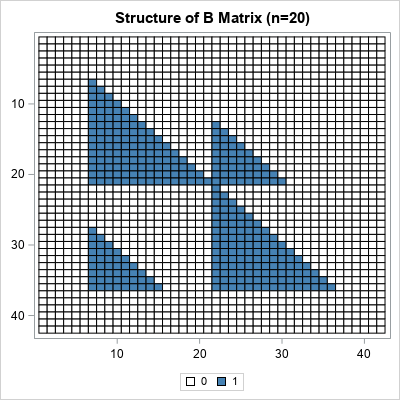The right-hand side of the linear system is also composed of binomial probabilities. The system is singular, but you can use a generalized inverse to obtain a solution. From the solution, you can compute the probability. For the details, see Facchinetti (2009).

Facchinetti includes a MATLAB program in the appendix of her paper. I converted the MATLAB program into SAS/IML and improved the efficiency by vectorizing some of the computations. You can download the SAS/IML program that computes the CDF of the Kolmogorov D statistic and all the graphs in this article. The name of the SAS/IML function is KolmogorovCDF, which requires two arguments, n (sample size) and D (value of the test statistic).

### Examples of using the CDF of the Kolmorov distribution

Suppose you have a sample of size 20. You can use a statistical table to look up the critical value of the Kolmogorov D statistic. For α = 0.05, the (two-sided) critical value for n=20 is D = 0.294. If you load and call the KolmogorovCDF function, you can confirm that the CDF for the Kolmogorov D distribution is very close to 0.95 when D=0.294:

```proc iml; load module=KolmogorovCDF; /* load the function that implements the Facchinetti method */ /* simple example of calling the function */ n = 20; D = 0.29408; cdf = KolmogorovCDF(n, D); print n D cdf;```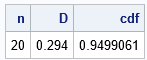The interpretation of this result is that if you draw a random samples of size 20 from the reference distribution, there is a 5% chance that the Kolmogorov D value will exceed 0.294. A test statistic that exceeds 0.294 implies that you should reject the null hypothesis at the 0.05 significance level.

### Visualize the Kolmogorov D distribution

If you can compute the CDF for one value of D, you can compute it for a sequence of D values. In this way, you can visualize the CDF curve. The following SAS/IML statements compute the CDF for the Kolmogorov D distribution when n=20 by running the computation on a sequence of D values in the open interval (0, 1):

```D = do(0.01, 0.99, 0.01); /* sequence of D values */ CDF = j(1, ncol(D), .); do i = 1 to ncol(D); CDF[i] = KolmogorovCDF(n, D[i]); /* CDF for each D */ end; title "Kolmogorov CDF, n=20"; call series(D, CDF) grid={x y}; /* create a graph of the CDF */```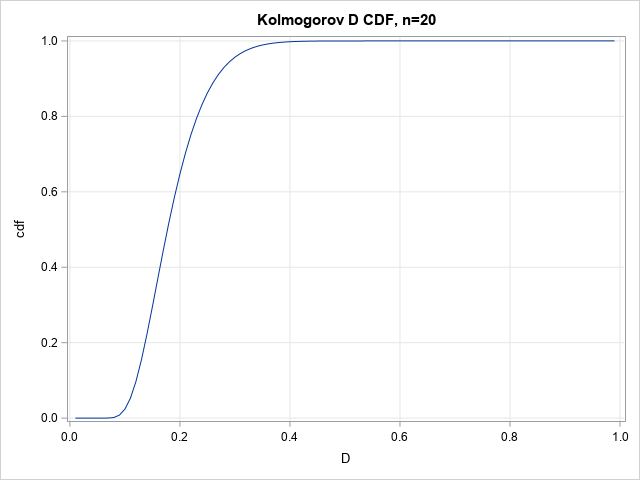The graph enables you to read probabilities and quantiles for the D20 distribution. The graph shows that almost all random samples of size 20 (drawn from the reference distribution) will have a D statistic of less than 0.4. Geometrically, the D statistic represents the largest gap between the empirical distribution and the reference distribution. A large gap indicates that the sample was probably not drawn from the reference distribution.

### Visualize a family of Kolmogorov D distributions

The previous graph was for n=20, but you can repeat the computation for other values of n to obtain a family of CDF curves for the Kolmogorov D statistic. (Facchinetti writes Dn to emphasize that the statistic depends on the sample size.) The computation is available in the program that accompanies this article. The results are shown below: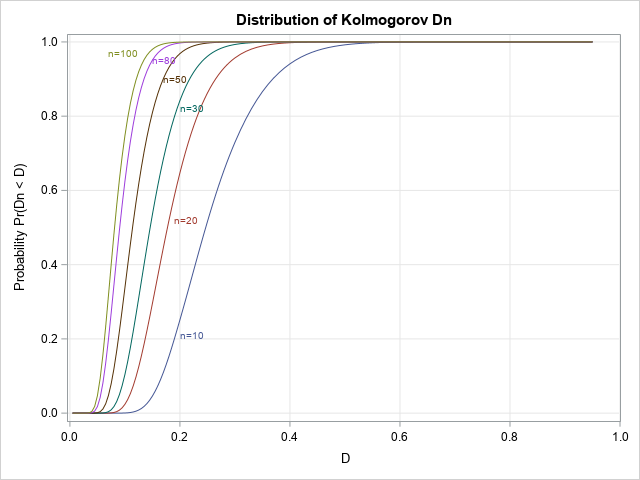This graph enables you to estimate probabilities and quantiles for several Dn distributions.

### Visualize a family of density curves

Because the PDF is the derivative of the CDF, you can use a forward difference approximation of the derivative to also plot the density of the distribution. The following density curves are derived from the previous CDF curves: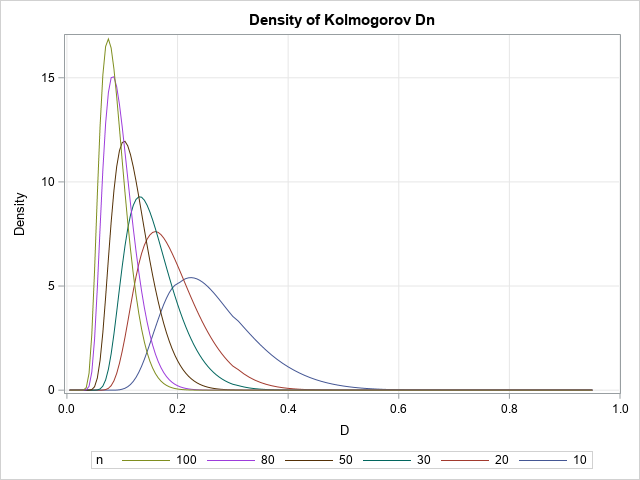### A table of critical values

If you can compute the CDF, you can compute quantiles by using the inverse CDF. You can use a root-finding technique to obtain a quantile.

In the SAS/IML language, the FROOT function can find univariate roots. The critical values of the Dn statistic are usually tabulated by listing the sample size down columns and the significance level (α) or confidence level (1-α) across the columns. Facchinetti (p. 352) includes a table of critical values in her paper. The following SAS/IML statements show how to recreate the table. For simplicity, I show only a subset of the table for n=20, 25, 20, and 35, and for four common values of α.

```/* A critical value is the root of the function f(D) = KolmogorovCDF(n, D) - (1-alpha) for any specified n and alpha value. */ start KolmogorovQuantile(D) global (n, alpha); return KolmogorovCDF(n, D) - (1-alpha); finish;   /* create a table of critical values for the following vector of n and alpha values */ nVals = do(20, 35, 5); alphaVals = {0.001 0.01 0.05 0.10}; CritVal = j(ncol(nVals), ncol(alphaVals), .); do i = 1 to ncol(nVals); n = nVals[i]; do j = 1 to ncol(alphaVals); alpha = alphaVals[j]; CritVal[i,j] = froot("KolmogorovQuantile", {0.01, 0.99}); end; end; print CritVal[r=nVals c=alphaVals format=7.5 L="Critical Values (n,alpha)"];```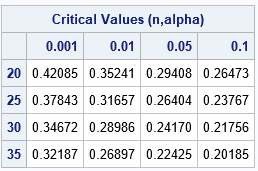For each sample size, the corresponding row of the table shows the critical values of D for which CDF(D) = 1-α. Of course, a printed table is unnecessary when you have a programmatic way to compute the quantiles.

### Summary

Facchinetti (2009) shows how to compute the CDF for the Kolmogorov D distribution for any value of n and D. The Kolmogorov D statistic is often used for goodness-of-fit tests. Facchinetti's method consists of forming and solving a system of linear equations. To make her work available to the SAS statistical programmer, I translated her MATLAB program into a SAS/IML program that computes the CDF of the Kolmogorov D distribution. This article demonstrates how to use the KolmogorovCDF in SAS/IML to compute and visualize the CDF and density of the Kolmogorov D statistic. It also shows how to use the computation in conjunction with a root-finding method to obtain quantiles and exact critical values of the Kolmogorov D distribution.

The post The Kolmogorov D distribution and exact critical values appeared first on The DO Loop.Sometimes in matrix computations, it is important to display the nonzero elements of a matrix. This can be useful for visualizing the structure of a sparse matrix (one that has many zeros) and it is also useful when describing a matrix algorithm (such as Gaussian elimination) that introduces zeros at each step of the algorithm.

Let A be the matrix you want to visualize. You can visualize the nonzero elements of the matrix A in a graph or in a table:

• Use a custom SAS format that prints an asterisk (*) for nonzero values and a blank for zero values.
• Create a discrete heat map of the binary matrix A^=0, which replaces the nonzero cells of A with the value 1.

### A text display method

For small matrices, you can use a custom SAS format to print the matrix. The format is defined by using PROC FORMAT. This format displays a star (*) for nonzero values and displays a blank for zero values:

```proc format; value SparseFmt 0 = " " /* display blank instead of 0 */ other = "*"; /* display '*' instead of number */ run;   proc iml; A = {1.0 0.2 0.0 0.0 0.0 0.0 1e-6, 0.2 1.0 0.2 0.0 0.0 0.0 0.0, 0.0 0.2 1.0 0.2 0.0 0.0 0.0, 0.0 0.0 0.2 1.0 0.2 0.0 0.0, 1e-6 0.0 0.0 0.2 1.0 0.2 0.0, 2e-6 1e-6 0.0 0.0 0.2 1.0 0.2, 3e-6 2e-6 1e-6 0.0 0.0 0.2 1.0 };   /* use a custom format to print * or blank in each cell */ print A[format=SparseFmt.];```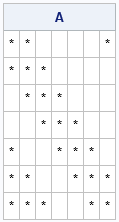You can see the banded structure of the matrix and also see that elements near the corners are nonzero. This textual visualization is concise and is used in textbooks about numerical linear algebra.

### A graphical method

For larger matrices, a graphical method is probably better. You can form the binary logical matrix B=(A^=0) and then plot B by using a two-color heat map. In the SAS/IML language, you can use the HEATMAPDISC call to create a discrete heat map of a matrix. I like to use white for the zeroes. The following SAS/IML statements define a 7 x 7 matrix and use a discrete heat map to display the nonzero values:

```/* discrete heat map of binary matrix A^=0 */ ods graphics / width=400px height=400px; call heatmapdisc(A^=0) colorramp={white steelblue} title="Discrete heat map of binary matrix";```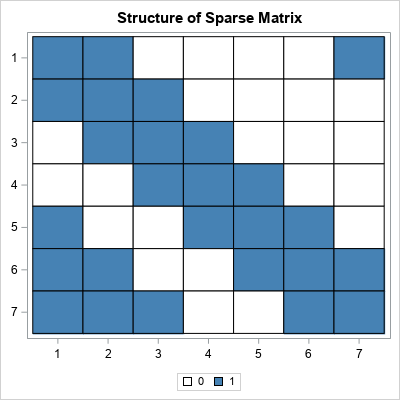The information in the heat map is identical to the printed display. However, for large matrices, the heat map is easier to view. As shown, you can also use the ODS GRAPHICS statement to control the dimensions of the graph. So, for example, if you are visualizing a square matrix, you can make the graph square.

The post Visualize the structure of a sparse matrix appeared first on The DO Loop.Rockin' around the Christmas tree
At the Christmas party hop.
– Brenda Lee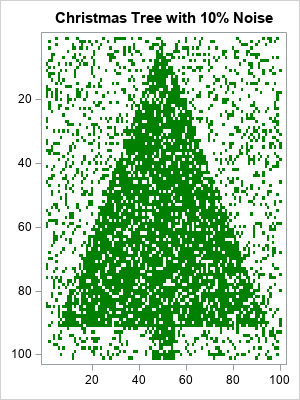Last Christmas, I saw a fun blog post that used optimization methods to de-noise an image of a Christmas tree. Although there are specialized algorithms that remove random noise from an image, I am not going to use a specialized de-noising algorithm. Instead, I will use the SVD algorithm, which is a general-purpose matrix algorithm that has many applications. One application is to construct a low-rank approximation of a matrix. This article applies the SVD algorithm to a noisy image of a Christmas tree. The result is recognizable as a Christmas tree, but much of the noise has been eliminated.

### A noisy Christmas tree image

The image to the right is a heat map of a binary (0/1) matrix that has 101 rows and 100 columns. First, I put the value 1 in certain cells so that the heat map resembles a Christmas tree. About 41% of the cells have the value 1. We will call these cells "the tree".

To add random noise to the image, I randomly switched 10% of the cells. The result is an image that is recognizable as a Christmas tree but is very noisy.

### Apply the SVD to a matrix

As mentioned earlier, I will attempt to de-noise the matrix (image) by using the general-purpose SVD algorithm, which is available in SAS/IML software. If M is the 101 x 100 binary matrix, then the following SAS/IML statements factor the matrix by using a singular-value decomposition. A series plot displays the first 20 singular values (ordered by magnitude) of the noisy Christmas tree matrix:

```call svd(U, D, V, M); /* A = U*diag(D)*V` */ call series(1:20, D) grid={x y} xvalues=1:nrow(D) label={"Component" "Singular Value"};```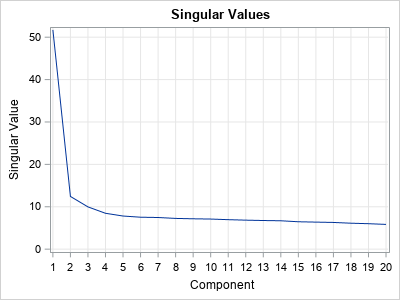The graph (which is similar to a scree plot in principal component analysis) indicates that the first four singular values are somewhat larger than the remainder. The following statements approximate M by using rank-4 matrix. The rank-4 matrix is not a binary matrix, but you can visualize the rank-4 approximation matrix by using a continuous heat map. For convenience, the matrix elements are shifted and scaled into the interval [0,1].

```keep = 4; /* how many components to keep? */ idx = 1:keep; Ak = U[ ,idx] * diag(D[idx]) * V[ ,idx]`; Ak = (Ak - min(Ak)) / range(Ak); /* for plotting, standardize into [0,1] */ s = "Rank " + strip(char(keep)) + " Approximation of Noisy Christmas Tree Image"; call heatmapcont(Ak) colorramp=ramp displayoutlines=0 showlegend=0 title=s range={0, 1};```### Apply a threshold cutoff to classify pixels

The continuous heat map shows a dark image of the Christmas tree surrounded by a light-green "fog". The dark-green pixels represent cells that have values near 1. The light-green pixels have smaller values. You can use a histogram to show the distribution of values in the rank-4 matrix:

```ods graphics / width=400px height=300px; call histogram(Ak);```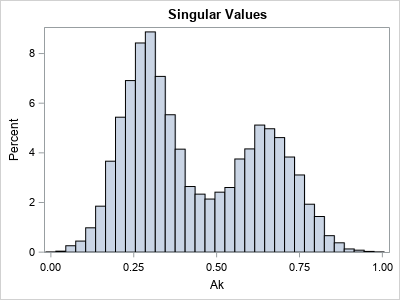You can think of the cell values as being a "score" that predicts whether each cell belongs to the Christmas tree (green) or not (white). The histogram indicates that the values in the matrix appear to be a mixture of two distributions. The high values in the histogram correspond to dark-green pixels (cells); the low values correspond to light-green pixels. To remove the light-green "fog" in the rank-4 image, you can use a "threshold value" to convert the continuous values to binary (0/1) values. Essentially, this operation predicts which cells are part of the tree and which are not.

For every choice of the threshold parameter, there will be correct and incorrect predictions:

• A "true positive" is a cell that belongs to the tree and is colored green.
• A "false positive" is a cell that does not belong to the tree but is colored green.
• A "true negative" is a cell that does not belong to the tree and is colored white.
• A "false negative" is a cell that belongs to the tree but is colored white.

By looking at the histogram, you might guess that a threshold value of 0.5 will do a good job of separating the low and high values. The following statements use 0.5 to convert the rank-4 matrix into a binary matrix, which you can think of as the predicted values of the de-noising process:

```t = 0.5; /* threshold parameter */ s = "Discrete Filter: Threshold = " + strip(char(t,4,2)) ; call HeatmapDisc(Ak > t) colorramp=ramp displayoutlines=0 showlegend=0 title=s;```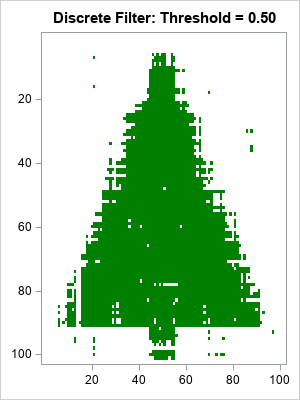Considering that 10% of the original image was corrupted, the heat map of the reconstructed matrix is impressive. It looks like a Christmas tree, but has much less noise. The reconstructed matrix agrees with the original (pre-noise) matrix for 98% of the cells. In addition:

• There are only a few false positives (green dots) that are far from the tree.
• There are some false negatives in the center of the tree, but many fewer than were in the original noisy image.
• The locations where the image is the most ragged is along the edge and trunk of the Christmas tree. In that region, the de-noising could not tell the difference between tree and non-tree.

### The effect of the threshold parameter

You might wonder what the reconstruction would look like for different choices of the cutoff threshold. The following statements create two other heat maps, one for the threshold value 0.4 and the other for the threshold value 0.6. As you might have guessed, smaller threshold values result in more false positives (green pixels away from the tree), whereas higher threshold values result in more false negatives (white pixels inside the tree).

```do t = 0.4 to 0.6 by 0.2; s = "Discrete Filter: Threshold = " + strip(char(t,4,2)) ; call HeatmapDisc(Ak > t) colorramp=ramp displayoutlines=0 showlegend=0 title=s; end;```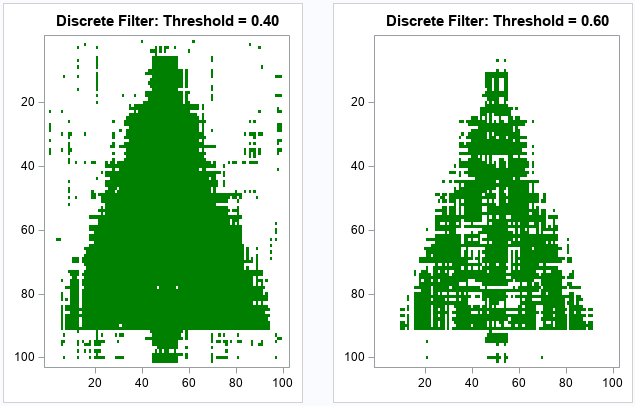### Summary

Although I have presented this experiment in terms of an image of a Christmas tree, it is really a result about matrices and low-rank approximations. I started with a binary 0/1 matrix, and I added noise to 10% of the cells. The SVD factorization enables you to approximate the matrix by using a rank-4 approximation. By using a cutoff threshold, you can approximate the original matrix before the random noise was added. Although the SVD algorithm is a general-purpose algorithm that was not designed for de-noising images, it does a good job eliminating the noise and estimating the original matrix.

If you are interested in exploring these ideas for yourself, you can download the complete SAS program that creates the graphs in this article.

The post Math-ing around the Christmas tree: Can the SVD de-noise an image? appeared first on The DO Loop.Binary matrices are used for many purposes. I have previously written about how to use binary matrices to visualize missing values in a data matrix. They are also used to indicate the co-occurrence of two events. In ecology, binary matrices are used to indicate which species of an animal are present in which ecological site. For example, if you remember your study of Darwin's finches in high school biology class, you might recall that certain finches (species) are present or absent on various Galapagos islands (sites). You can use a binary matrix to indicate which finches are present on which islands.

Recently I was involved in a project that required performing a permutation test on rows of a binary matrix. As part of the project, I had to solve three smaller problems involving rows of a binary matrix:

1. Given two rows, find the locations at which the rows differ.
2. Given two binary matrices, determine how similar the matrices are by computing the proportion of elements that are the same.
3. Given two rows, swap some of the elements that differ.

This article shows how to solve each problem by using the SAS/IML matrix language. A future article will discuss permutation tests for binary matrices. For clarity, I introduce the following macro that uses a temporary variable to swap two sets of values:

```/* swap values of A and B. You can use this macro in the DATA step or in the SAS/IML language */ %macro SWAP(A, B, TMP=_tmp); &TMP = &A; &A = &B; &B = &TMP; %mend;```

### Where do binary matrices differ?

The SAS/IML matrix language enables you to treat matrices as high-level objects. You often can answer questions about matrices without writing loops that iterate over the elements of the matrices. For example, if you have two matrices of the same dimensions, you can determine the cells at which the matrices are unequal by using the "not equal" (^=) operator. The following SAS/IML statements define two 2 x 10 matrices and use the "not equal" operator to find the cells that are different:

```proc iml; A = {0 0 1 0 0 1 0 1 0 0 , 1 0 0 0 0 0 1 1 0 1 }; B = {0 0 1 0 0 0 0 1 0 1 , 1 0 0 0 0 1 1 1 0 0 }; colLabel = "Sp1":("Sp"+strip(char(ncol(A)))); rowLabel = "A1":("A"+strip(char(nrow(A))));   /* 1. Find locations where binary matrices differ */ Diff = (A^=B); print Diff[c=colLabel r=rowLabel];```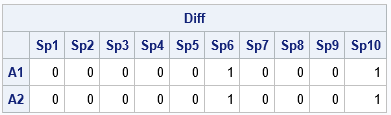The matrices A and B are similar. They both have three 1s in the first row and fours 1s in the second row. However, the output shows that the matrices are different for the four elements in the sixth and tenth columns. Although I used entire matrices for this example, the same operations work on row vectors.

### The proportion of elements in common

You can use various statistics to measure the similarity between the A and B matrices. A simple statistic is the proportion of elements that are in common. These matrices have 20 elements, and 16/20 = 0.8 are common to both matrices. You can compute the proportion in common by using the express (A=B)[:], or you can use the following statements if you have previously computed the Diff matrix:

```/* 2. the proportion of elements in B that are the same as in A */ propDiff = 1 - Diff[:]; print propDiff;```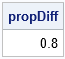As a reminder, the mean subscript reduction operator ([:]) computes the mean value of the elements of the matrix. For a binary matrix, the mean value is the proportion of ones.

### Swap elements of rows

The first two tasks were easy. A more complicated task is swapping values that differ between rows. The swapping operation is not difficult, but it requires finding the k locations where the rows differ and then swapping all or some of those values. In a permutation test, the number of elements that you swap is a random integer between 1 and k, but for simplicity, this example uses the SWAP macro to swap two cells that differ. For clarity, the following example uses temporary variables such as x1, x2, d1, and d2 to swap elements in the matrix A:

```/* specify the rows whose value you want to swap */ i1 = 1; /* index of first row to compare and swap */ i2 = 2; /* index of second row to compare and swap */   /* For clarity, use temp vars x1 & x2 instead of A[i1, ] and A[i2, ] */ x1 = A[ i1, ]; /* get one row */ x2 = A[ i2, ]; /* get another row */ idx = loc( x1^=x2 ); /* find the locations where rows differ */ if ncol(idx) > 0 then do; /* do the rows differ? */ d1 = x1[ ,idx]; /* values at the locations that differ */ d2 = x2[ ,idx]; print (d1//d2)[r={'r1' 'r2'} L='Original'];   /* For a permutation test, choose a random number of locations to swap */ /* numSwaps = randfun(1, "Integer", 1, n); idxSwap = sample(1:ncol(idx), numSwaps, "NoReplace"); */ idxSwap = {2 4}; /* for now, hard-code locations to swap */ %SWAP(d1[idxSwap], d2[idxSwap]); print (d1//d2)[r={'d1' 'd2'} L='New']; /* update matrix */ A[ i1, idx] = d1; A[ i2, idx] = d2; end; print A[c=colLabel r=rowLabel];```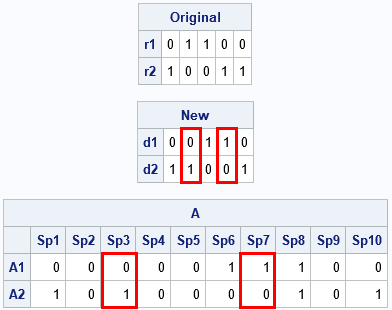The vectors x1 and x2 are the rows of A to compare. The vectors d1 and d2 are the subvectors that contain only the elements of x1 and x2 that differ. The example swaps the second and fourth columns of d1 and d2. The new values are then inserted back into the matrix A. You can compare the final matrix to the original to see that the process swapped two elements in each of two rows.

Although the examples are for binary matrices, these techniques work for any numerical matrices.

The post Swap elements in binary matrices appeared first on The DO Loop.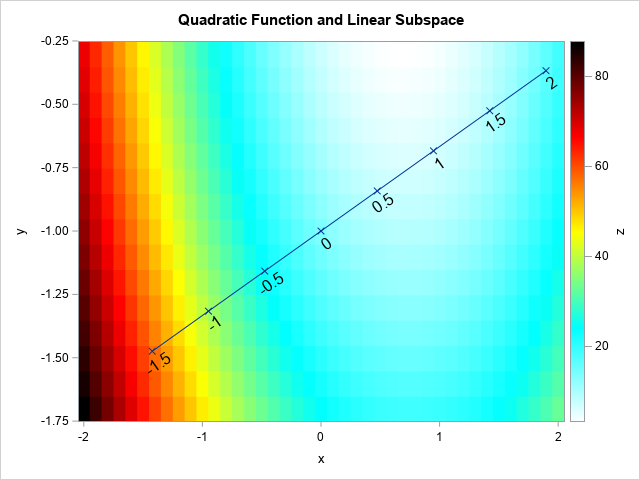This article discusses how to restrict a multivariate function to a linear subspace. This is a useful technique in many situations, including visualizing an objective function that is constrained by linear equalities. For example, the graph to the right is from a previous article about how to evaluate quadratic polynomials. The graph shows a heat map for a quadratic polynomial of two variables. The diagonal line represents a linear constraint between the X and Y variables. If you restrict the polynomial to the diagonal line, you obtain a one-dimensional function that you can easily graph and visualize. By repeating this process for other lines, you can construct a "stack" of lower-dimensional slices that enable you to understand the graph of the original bivariate function.

This example generalizes. For a function of many variables, you can restrict the function to a lower-dimensional subspace. By visualizing the restricted function, you can understand the high-dimensional function better. I previously demonstrated this technique for multidimensional regression models by using the SLICEFIT option in the EFFECTPLOT statement in SAS. This article shows how to use ideas from vector calculus to restrict a function to a parameterized linear subspace.

### Visualize high-dimensional data and functions

There are basically two techniques for visualizing high-dimensional objects: projection and slicing. Many methods in multivariate statistics compute a linear subspace such that the projection of the data onto the subspace has a desirable property. One example is principal component analysis, which endeavors to find a low dimensional subspace that captures most of the variation in the data. Another example is linear discriminant analysis, which aims to find a linear subspace that maximally separates groups in the data. In both cases, the data are projected onto the computed subspaces to reveal characteristics of the data. There are also statistical methods that attempt to find nonlinear subspaces.

Whereas projection is used for data, slicing is often used to visualize the graphs of functions. In regression, you model a response variable as a function of the explanatory variables. Slicing the function along a linear subspace of the domain can reveal important characteristics about the function. That is the method used by the SLICEFIT option in the EFFECTPLOT statement, which enables you to visualize multidimensional regression models. The most common "slice" for a regression model is to specify constant values for all but one continuous variable in the model.

When visualizing an objective function that is constrained by a set of linear equalities, the idea is similar. The main difference is that the "slice" is usually determined by a general linear combination of variables.

### Restrict a function to a one-dimensional subspace

A common exercise in multivariate calculus is to restrict a bivariate function to a line. (This is equivalent to a "slice" of the bivariate function.) Suppose that you choose a point, x0, and a unit vector, u, which represents the "direction". Then a parametric line through x0 in the direction of u is given by the vector expression v(t) = x0 + t u, where t is any real number. If you restrict a multivariate function to the image of v, you obtain a one-dimensional function.

For example, consider the quadratic polynomial in two variables:
f(x,y) = (9*x##2 + x#y + 4*y##2) - 12*x - 4*y + 6
The function is visualized by the heat map at the top of this article. Suppose x0 = {0, -1} and choose u to be the unit vector in the direction of the vector {3, 1}. (This choice corresponds to a linear constraint of the form x – 3y = 3.)

The following SAS/IML program constructs the parameterized line v(t) for a uniform set of t values. The quadratic function is evaluated at this set of values and the resulting one-dimensional function is graphed:

```proc iml; /* Evaluate the quadratic function at each column of X and return a row vector. */ start Func(XY); x = XY[1,]; y = XY[2,]; return (9*x##2 + x#y + 4*y##2) - 12*x - 4*y + 6; finish;   x0 = {0, -1}; /* evaluate polynomial at this point */ d = {3, 1}; /* vector that determines direction */ u = d / norm(d); /* unit vector (direction) */   t = do(-2, 2, 0.1); /* parameter values */ v = x0 + t @ u; /* evenly spaced points along the linear subspace */ f = Func(v); /* evaluate the 2-D function along the 1-D subspace */ title "Quadratic Form Evaluated on Linear Subspace"; call series(t, f) grid={x y};```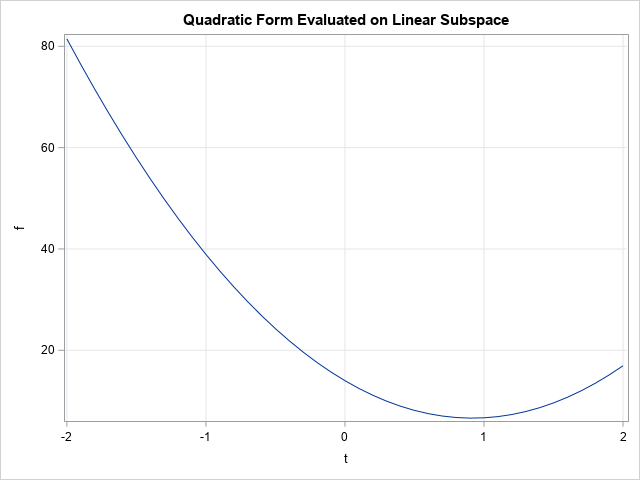The graph shows the restriction of the 2-D function to the 1-D subspace. According to the graph, the restricted function reaches a minimum value for t ≈ 0.92. The corresponding (x, y) values and the corresponding function value are shown below:

```tMin = 0.92; /* t* = parameter near the minimum */ vMin = x0 + tMin * u; /* corresponding (x(t*), y(t*)) */ fMin = Func(vMin); /* f(x(t*), y(t*)) */ print tMin vMin fMin;```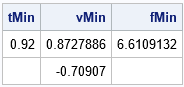If you look back to the heat map at the top of this article, you will see that the value (x,y) = (0.87,-0.71) correspond to the location for which the function achieves a minimum value when restricted to the linear subspace. The value of the function at that (x,y) value is approximately 6.6.

The SAS/IML program uses the Kronecker direct-product operator (@) to create points along the line. The operation is v = x0 + t @ u. The symbols x0 and u are both column vectors with two elements. The Kronecker product creates a 2 x k matrix, where k is the number of elements in the row vector t. The Kronecker product enables you to vectorize the program instead of looping over the elements of t and forming the individual vectors x0 + t[i]*u.

You can generalize this example to evaluate a multivariate function on a two-dimensional subspace. If u1 and u2 are two orthogonal, p-dimensional, unit vectors, the expression x0 + s*u1 + t*u2 spans a two-dimensional subspace of Rp. You can use the ExpandGrid function in SAS/IML to generate ordered pairs (s, t) on a two-dimensional grid.

### Summary

In summary, you can slice a multivariate function along a linear subspace. Usually, 1-D or 2-D subspaces are used and the resulting (restricted) function is graphed. This helps you to understand the function. You can choose a series of slices (often parallel to each other) and "stack" the slices in order to visualize the multivariate function. Typically, this technique works best for functions that have three or four continuous variables.

The post Evaluate a function on a linear subspace appeared first on The DO Loop.What is an efficient way to evaluate a multivariate quadratic polynomial in p variables? The answer is to use matrix computations! A multivariate quadratic polynomial can be written as the sum of a purely quadratic term (degree 2), a purely linear term (degree 1), and a constant term (degree 0). The purely quadratic term is called a quadratic form. This article shows how to use matrix computations to efficiently evaluate a multivariate quadratic polynomial.

### Quadratic polynomials and matrix expressions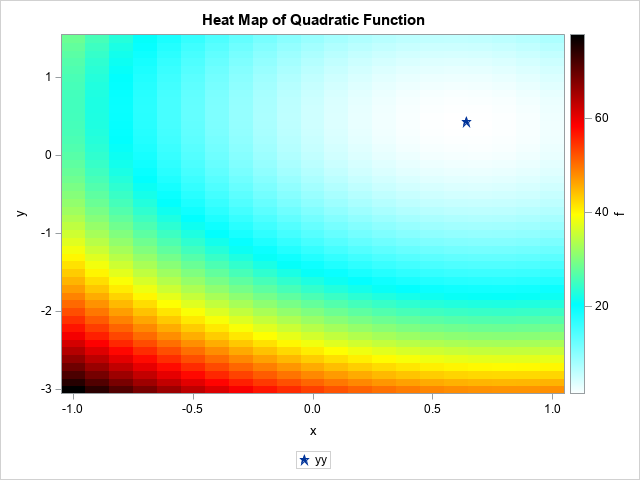As I wrote in a previous article about optimizing a quadratic function, the matrix of second derivatives and the gradient of first derivatives appear in the matrix representation of a quadratic polynomial. I will use the same example as in my previous article. Namely, the following quadratic polynomial in two variables:
f(x,y) = (9*x##2 + x#y + 4*y##2) - 12*x - 4*y + 6
= (1/2) x` Q x + L` x + 6
where Q = {18  1, 1  8} is a 2x2 symmetric matrix, L = {-12  -4} is a column vector, and x = {x, y} is a column vector that represents the coordinates at which to evaluate the quadratic function.

The graph at the right visualizes this quadratic function by using a heat map. Small values of the function are shown in white. Larger values of the function are shown in blues and greens. The largest values are shown in reds and blacks. The global minimum of this function is approximately (x, y) = (0.64, 0.42), and that point is indicated by a star.

This example generalizes. Every quadratic function in p variables can be written as the sum of a quadratic form (1/2 x` Q x), a linear term (L` x) and a constant. Here Q is a p x p symmetric matrix of second derivatives (the Hessian matrix of f) and L and x are p-dimensional column vectors.

### Evaluate quadratic polynomial in SAS

Because the computation involves vectors and matrices, the SAS/IML language is the natural place to use matrices to evaluate a quadratic function. The following SAS/IML statements implement a simple function to evaluate a quadratic polynomial (given in terms of Q, L, and a constant) at an arbitrary two-dimensional vector, x:

```proc iml; /* Evaluate f(x) = 0.5 * x` * Q * x + L`*x + const, where Q is p x p symmetric matrix, L and x are col vector with p elements. This version evaluates ONE vector x and returns a scalar value. */ start EvalQuad(x, Q, L, const=0); return 0.5 * x`*Q*x + L`*x + const; finish;   /* compute Q and L for f(x,y)= 9*x##2 + x#y + 4*y##2) - 12*x - 4*y + 6 */ Q = {18 1, /* matrix of second derivatives */ 1 8}; L = { -12, -4}; /* use column vectors for L and x */ const = 6; x0 = {0, -1}; /* evaluate polynomial at this point */ f = EvalQuad(x0, Q, L, const); print f[L="f(0,-1)"];```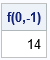### Evaluate a quadratic polynomial at multiple points

When I implement a function in the SAS/IML language, I try to "vectorize" it so that it can evaluate multiple points in a single call. Often you can use matrix operations to vectorize a function evaluation, but I don't see how to make the math work for this problem. The natural way to evaluate a quadratic polynomial at k vectors X1, X2, ..., Xk, is to pack those vectors into a p x k matrix X such that each column of X is a point at which to evaluate the polynomial. Unfortunately, the matrix computation of the quadratic form M = 0.5 * X`*Q*X results in a k x k matrix. Only the k diagonal elements are needed for evaluating the polynomial on the k input vectors, so although it is possible to compute M, doing so would be very inefficient.

In this case, it seems more efficient to loop over the columns of X. The following function implements a SAS/IML module that evaluates a quadratic polynomial at every column of X and returns a row vector of the results. The module is demonstrated by calling it on a matrix that has 5 columns.

```/* Evaluate the quadratic function at each column of X and return a row vector. */ start EvalQuadVec(X, Q, L, const=0); f = j(1, ncol(X), .); do i = 1 to ncol(X); v = X[,i]; f[i] = 0.5 * v`*Q*v + L`*v + const; end; return f; finish;   /* X1 X2 X3 X4 X5 */ vx = {-1 -0.5 0 0.5 1 , -3 -2 -1 0 1 }; f = EvalQuadVec(vx, Q, L, const=0); print (vx // f)[r={'x' 'y' 'f(x,y)'} c=('X1':'X5')];```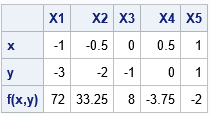### Evaluate a quadratic polynomial on a uniform grid of points

You can use the EvalQuadVec function to evaluate a quadratic polynomial on any set of multiple points. In particular, you can use the ExpandGrid function to construct a regular 2-D grid of points. By evaluating the function at each point on the grid, you can visualize the function. The following statements create a heat map of the function on a regular grid. The heat map is shown at the top of this article.

```x = do(-1, 1, 0.1); y = do(-3, 1.5, 0.1); xy = expandgrid(x, y); /* 966 x 2 matrix */ f = EvalQuadVec(xy`, Q, L, const); /* evaluate polynomial at all points */   /* write results to a SAS data set and visualize the function by using a heat map */ M = xy || f`; create Heatmap from M[c={'x' 'y' 'f'}]; append from M; close; QUIT;   data optimal; xx=0.64; yy=0.42; /* optional: add the optimal (x,y) value */ run; data All; set Heatmap optimal; run;   title "Heat Map of Quadratic Function"; proc sgplot data=All; heatmapparm x=x y=y colorresponse=f / colormodel= (WHITE CYAN YELLOW RED BLACK); scatter x=xx y=yy / markerattrs=(symbol=StarFilled); xaxis offsetmin=0 offsetmax=0; yaxis offsetmin=0 offsetmax=0; run;```

Perhaps you do not often use quadratic polynomials. This technique is useful even for general nonlinear functions because it enables you to find the best quadratic approximation to a multivariate function at any point x0. The multivariate Taylor series at the point x0, truncated at second order, is
f(x) ≈ f(x0) + L` · (xx0) + (1/2) (xx0)` · Q · (xx0)
where L = ∇f(x0) is the gradient of f evaluate at x0 and Q = D2f(x0) is the symmetric Hessian matrix of second derivatives of f evaluated at x0.

### Summary

In summary, you can use matrix computations to evaluate a multivariate quadratic polynomial. This article shows how to evaluate a quadratic polynomial at multiple points. For a polynomial of two variables, you can use this technique to visualize quadratic functions.

The post Evaluate a quadratic polynomial in SAS appeared first on The DO Loop.Biplots are two-dimensional plots that help to visualize relationships in high dimensional data. A previous article discusses how to interpret biplots for continuous variables. The biplot projects observations and variables onto the span of the first two principal components. The observations are plotted as markers; the variables are plotted as vectors. The observations and/or vectors are not usually on the same scale, so they need to be rescaled so that they fit on the same plot. There are four common scalings (GH, COV, JK, and SYM), which are discussed in the previous article.

This article shows how to create biplots in SAS. In particular, the goal is to create the biplots by using modern ODS statistical graphics. You can obtain biplots that use the traditional SAS/GRAPH system by using the %BIPLOT macro by Michael Friendly. The %BIPLOT macro is very powerful and flexible; it is discussed later in this article.

There are four ways to create biplots in SAS by using ODS statistical graphics:

• You can use PROC PRINQUAL in SAS/STAT software to create the COV biplot.
• If you have a license for SAS/GRAPH software (and SAS/IML software), you can use Friendly's %BIPLOT macro and use the OUT= option in the macro to save the coordinates of the markers and vectors. You can then use PROC SGPLOT to create a modern version of Friendly's biplot.
• You can use the matrix computations in SAS/IML to "manually" compute the coordinates of the markers and vectors. (These same computations are performed internally by the %BIPLOT macro.) You can use the Biplot module to create a biplot, or you can use the WriteBiplot module to create a SAS data set that contains the biplot coordinates. You can then use PROC SGPLOT to create the biplot.

For consistency with the previous article, all methods in this article standardize the input variables to have mean zero and unit variance (use the SCALE=STD option in the %BIPLOT macro). All biplots show projections of the same four-dimensional Fisher's iris data. The following DATA step assigns a blank label. If you do not supply an ID variable, some biplots display observations numbers.

```data iris; set Sashelp.iris; id = " "; /* create an empty label variable */ run;```

### Use PROC PRINQUAL to compute the COV biplot

The PRINQUAL procedure can perform a multidimensional preference analysis, which is visualized by using a MDPREF plot. The MDPREF plot is closely related to biplot (Jackson (1991), A User’s Guide to Principal Components, p. 204). You can get PROC PRINQUAL to produce a COV biplot by doing the following:

• Use the N=2 option to specify you want to compute two principal components.
• Use the MDPREF=1 option to specify that the procedure should not rescale the vectors in the biplot. By default, MDPREF=2.5 and the vectors appear 2.5 larger than they should be. (More on scaling vectors later.)
• Use the IDENTITY transformation so that the variables are not transformed in a nonlinear manner.

The following PROC PRINQUAL statements produce a COV biplot (click to enlarge):

```proc prinqual data=iris plots=(MDPref) n=2 /* project onto Prin1 and Prin2 */ mdpref=1; /* use COV scaling */ transform identity(SepalLength SepalWidth PetalLength PetalWidth); /* identity transform */ id ID; ods select MDPrefPlot; run;```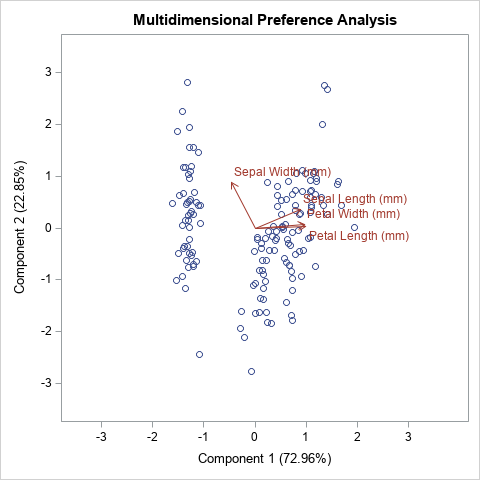### Use Friendly's %BIPLOT macro

Friendly's books [SAS System for Statistical Graphics (1991) and Visualizing Categorical Data (2000)] introduced many SAS data analysts to the power of using visualization to accompany statistical analysis, and especially the analysis of multivariate data. His macros use traditional SAS/GRAPH graphics from the 1990s. In the mid-2000s, SAS introduced ODS statistical graphics, which were released with SAS 9.2. Although the %BIPLOT macro does not use ODS statistical graphics directly, the macro supports the OUT= option, which enables you to create an output data set that contains all the coordinates for creating a biplot.

The following example assumes that you have downloaded the %BIPLOT macro and the %EQUATE macro from Michael Friendly's web site.

```/* A. Use %BIPLOT macro, which uses SAS/IML to compute the biplot coordinates. Use the OUT= option to get the coordinates for the markers and vectors. B. Transpose the data from long to wide form. C. Use PROC SGPLOT to create the biplot */ %let FACTYPE = SYM; /* options are GH, COV, JK, SYM */ title "Biplot: &FACTYPE, STD"; %biplot(data=iris, var=SepalLength SepalWidth PetalLength PetalWidth, id=id, factype=&FACTYPE, /* GH, COV, JK, SYM */ std=std, /* NONE, MEAN, STD */ scale=1, /* if you do not specify SCALE=1, vectors are auto-scaled */ out=biplotFriendly,/* write SAS data set with results */ symbols=circle dot, inc=1);   /* transpose from long to wide */ data Biplot; set biplotFriendly(where=(_TYPE_='OBS') rename=(dim1=Prin1 dim2=Prin2 _Name_=_ID_)) biplotFriendly(where=(_TYPE_='VAR') rename=(dim1=vx dim2=vy _Name_=_Variable_)); run;   proc sgplot data=Biplot aspect=1 noautolegend; refline 0 / axis=x; refline 0 / axis=y; scatter x=Prin1 y=Prin2 / datalabel=_ID_; vector x=vx y=vy / datalabel=_Variable_ lineattrs=GraphData2 datalabelattrs=GraphData2; xaxis grid offsetmin=0.1 offsetmax=0.2; yaxis grid; run;```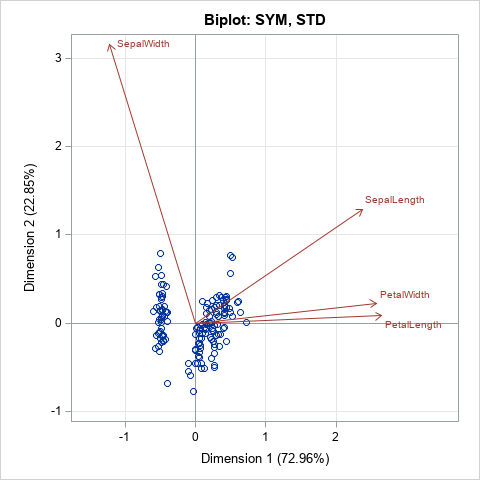Because you are using PROC SGPLOT to display the biplot, you can easily configure the graph. For example, I added grid lines, which are not part of the output from the %BIPLOT macro. You could easily change attributes such as the size of the fonts or add additional features such as an inset. With a little more work, you can merge the original data and the biplot data and color-code the markers by a grouping variable (such as Species) or by a continuous response variable.

Notice that the %BUPLOT macro supports a SCALE= option. The SCALE= option applies an additional linear scaling to the vectors. You can use this option to increase or decrease the lengths of the vectors in the biplot. For example, in the SYM biplot, shown above, the vectors are long relative to the range of the data. If you want to display vectors that are only 25% as long, you can specify SCALE=0.25. You can specify numbers greater than 1 to increase the vector lengths. For example, SCALE=2 will double the lengths of the vectors. If you omit the SCALE= option or set SCALE=0, then the %BIPLOT macro automatically scales the vectors to the range of the data. If you use the SCALE= option, you should tell the reader that you did so.

### SAS/IML modules that compute biplots

The %BIPLOT macro uses SAS/IML software to compute the locations of the markers and vectors for each type of biplot. I wrote three SAS/IML modules that perform the three steps of creating a biplot:

• The CalcBiplot module computes the projections of the observations and scores onto the first few principal components. This module (formerly named CalcPrinCompBiplot) was written in the mid-2000s and has been distributed as part of the SAS/IML Studio application. It returns the scores and vectors as SAS/IML matrices.
• The WriteBiplot module calls the CalcBiplot module and then writes the scores to a SAS data set called _SCORES and the vectors (loadings) to a SAS data set called _VECTORS. It also creates two macro variables, MinAxis and MaxAxis, which you can use if you want to equate the horizontal and vertical scales of the biplot axes.
• The Biplot function calls the WriteBiplot module and then calls PROC SGPLOT to create a biplot. It is the "raw SAS/IML" version of the %BIPLOT macro.

You can use the CalcBiplot module to compute the scores and vectors and return them in IML matrices. You can use the WriteBiplot module if you want that information in SAS data sets so that you can create your own custom biplot. You can use the Biplot module to create standard biplots. The Biplot and WriteBiplot modules are demonstrated in the next sections.

### Use the Biplot module in SAS/IML

The syntax of the Biplot module is similar to the %BIPLOT macro for most arguments. The input arguments are as follows:

• X: The numerical data matrix
• ID: A character vector of values used to label rows of X. If you pass in an empty matrix, observation numbers are used to label the markers. This argument is ignored if labelPoints=0.
• varNames: A character vector that contains the names of the columns of X.
• FacType: The type of biplot: 'GH', 'COV', 'JK', or 'SYM'.
• StdMethod: How the original variables are scaled: 'None', 'Mean', or 'Std'.
• Scale: A numerical scalar that specifies additional scaling applied to vectors. By default, SCALE=1, which means the vectors are not scaled. To shrink the vectors, specify a value less than 1. To lengthen the vectors, specify a value greater than 1. (Note: The %BIPLOT macro uses SCALE=0 as its default.)
• labelPoints: A binary 0/1 value. If 0 (the default) points are not labeled. If 1, points are labeled by the ID values. (Note: The %BIPLOT macro always labels points.)

The last two arguments are optional. You can specify them as keyword-value pairs outside of the parentheses. The following examples show how you can call the Biplot module in a SAS/IML program to create a biplot:

```ods graphics / width=480px height=480px; proc iml; /* assumes the modules have been previously stored */ load module=(CalcBiplot WriteBiplot Biplot); use sashelp.iris; read all var _NUM_ into X[rowname=Species colname=varNames]; close;   title "COV Biplot with Scaled Vectors and Labels"; run Biplot(X, Species, varNames, "COV", "Std") labelPoints=1; /* label obs */   title "JK Biplot: Relationships between Observations"; run Biplot(X, NULL, varNames, "JK", "Std");   title "JK Biplot: Automatic Scaling of Vectors"; run Biplot(X, NULL, varNames, "JK", "Std") scale=0; /* auto scale; empty ID var */   title "SYM Biplot: Vectors Scaled by 0.25"; run Biplot(X, NULL, varNames, "SYM", "Std") scale=0.25; /* scale vectors by 0.25 */```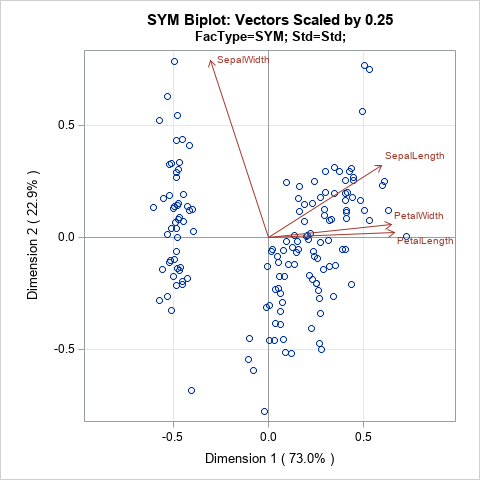The program creates four biplots, but only the last one is shown. The last plot uses the SCALE=0.25 option to rescale the vectors of the SYM biplot. You can compare this biplot to the SYM biplot in the previous section, which did not rescale the length of the vectors.

### Use the WriteBiplot module in SAS/IML

If you prefer to write an output data set and then create the biplot yourself, use the WriteBiplot module. After loading the modules and the data (see the previous section), you can write the biplot coordinates to the _Scores and _Vectors data sets, as follows. A simple DATA step appends the two data sets into a form that is easy to graph:

```run WriteBiplot(X, NULL, varNames, "JK", "Std") scale=0; /* auto scale vectors */ QUIT;   data Biplot; set _Scores _Vectors; /* append the two data sets created by the WriteBiplot module */ run;   title "JK Biplot: Automatic Scaling of Vectors"; title2 "FacType=JK; Std=Std"; proc sgplot data=Biplot aspect=1 noautolegend; refline 0 / axis=x; refline 0 / axis=y; scatter x=Prin1 y=Prin2 / ; vector x=vx y=vy / datalabel=_Variable_ lineattrs=GraphData2 datalabelattrs=GraphData2; xaxis grid offsetmin=0.1 offsetmax=0.1 min=&minAxis max=&maxAxis; yaxis grid min=&minAxis max=&maxAxis; run;```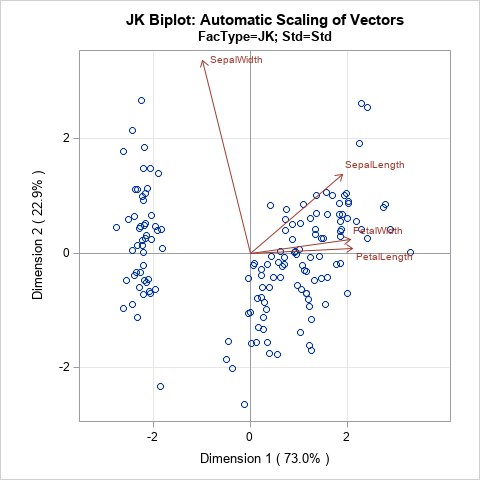In the program that accompanies this article, there is an additional example in which the biplot data is merged with the original data so that you can color-code the observations by using the Species variable.

### Summary

This article shows four ways to use modern ODS statistical graphics to create a biplot in SAS. You can create a COV biplot by using the PRINQUAL procedure. If you have a license for SAS/IML and SAS/GRAPH, you can use Friendly's %BIPLOT macro to write the biplot coordinates to a SAS data set, then use PROC SGPLOT to create the biplot. This article also presents SAS/IML modules that compute the same biplots as the %BIPLOT macro. The WriteBiplot module writes the data to two SAS data sets (_Score and _Vector), which can be appended and used to plot a biplot. This gives you complete control over the attributes of the biplot. Or, if you prefer, you can use the Biplot module in SAS/IML to automatically create biplots that are similar to Friendly's but are displayed by using ODS statistical graphics.

You can download the complete SAS program that is used in this article. For convenience, I have also created a separate file that defines the SAS/IML modules that create biplots.

The post Create biplots in SAS appeared first on The DO Loop.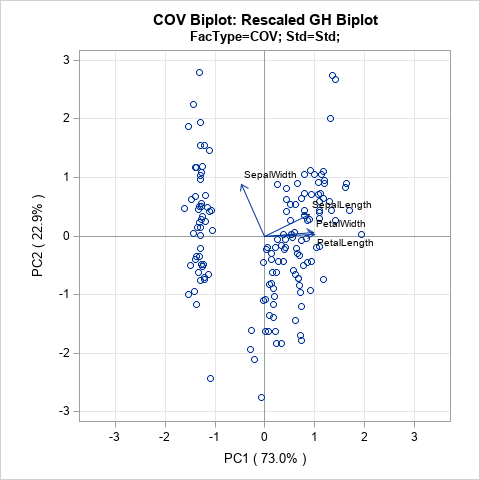Principal component analysis (PCA) is an important tool for understanding relationships in multivariate data. When the first two principal components (PCs) explain a significant portion of the variance in the data, you can visualize the data by projecting the observations onto the span of the first two PCs. In a PCA, this plot is known as a score plot. You can also project the variable vectors onto the span of the PCs, which is known as a loadings plot. See the article "How to interpret graphs in a principal component analysis" for a discussion of the score plot and the loadings plot.

A biplot overlays a score plot and a loadings plot in a single graph. An example is shown at the right. Points are the projected observations; vectors are the projected variables. If the data are well-approximated by the first two principal components, a biplot enables you to visualize high-dimensional data by using a two-dimensional graph.

In general, the score plot and the loadings plot will have different scales. Consequently, you need to rescale the vectors or observations (or both) when you overlay the score and loadings plots. There are four common choices of scaling. Each scaling emphasizes certain geometric relationships between pairs of observations (such as distances), between pairs of variables (such as angles), or between observations and variables. This article discusses the geometry behind two-dimensional biplots and shows how biplots enable you to understand relationships in multivariate data.

Some material in this blog post is based on documentation that I wrote in 2004 when I was working on the SAS/IML Studio product and writing the SAS/IML Studio User's Guide. The documentation is available online and includes references to the literature.

### The Fisher iris data

A previous article shows the score plot and loadings plot for a PCA of Fisher's iris data. For these data, the first two principal components explain 96% of the variance in the four-dimensional data. Therefore, these data are well-approximated by a two-dimensional set of principal components. For convenience, the score plot (scatter plot) and the loadings plot (vector plot) are shown below for the iris data. Notice that the loadings plot has a much smaller scale than the score plot. If you overlay these plots, the vectors would appear relatively small unless you rescale one or both plots.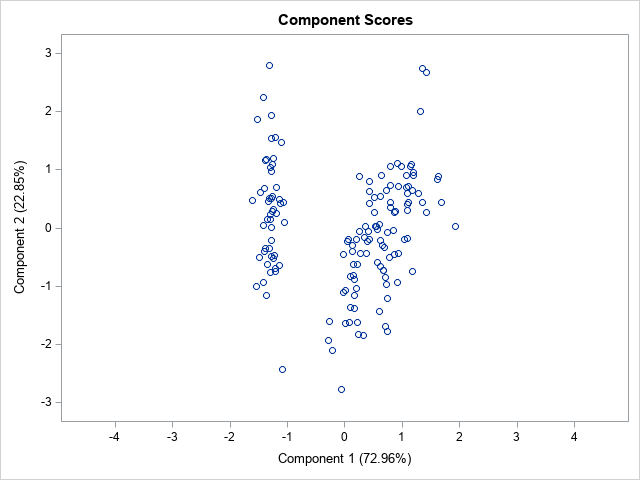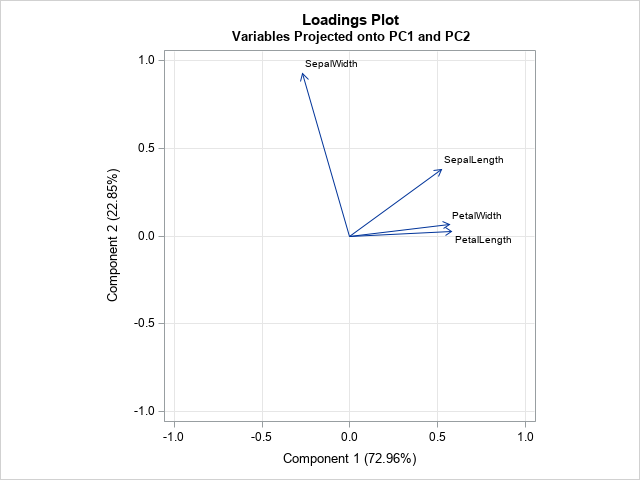### The mathematics of the biplot

You can perform a PCA by using a singular value decomposition of a data matrix that has N rows (observations) and p columns (variables). The first step in constructing a biplot is to center and (optionally) scale the data matrix. When variables are measured in different units and have different scales, it is usually helpful to standardize the data so that each column has zero mean and unit variance. The examples in this article use standardized data.

The heart of the biplot is the singular value decomposition (SVD). If X is the centered and scaled data matrix, then the SVD of X is
X = U L V`
where U is an N x N orthogonal matrix, L is a diagonal N x p matrix, and V is an orthogonal p x p matrix. It turns out that the principal components (PCs) of X`X are the columns of V and the PC scores are the columns of U. If the first two principal components explain most of the variance, you can choose to keep only the first two columns of U and V and the first 2 x 2 submatrix of L. This is the closest rank-two approximation to X. In a slight abuse of notation,
X ≈ U L V`
where now U, L, and V all have only two columns.

Since L is a diagonal matrix, you can write L = Lc L1-c for any number c in the interval [0, 1]. You can then write
X ≈ (U Lc)(L1-c V`)
= A B

This the factorization that is used to create a biplot. The most common choices for c are 0, 1, and 1/2.

### The four types of biplots

The choice of the scaling parameter, c, will linearly scale the observations and vectors separately. In addition, you can write X ≈ (β A) (B / β) for any constant β. Each choice for c corresponds to a type of biplot:

• When c=0, the vectors are represented faithfully. This corresponds to the GH biplot. If you also choose β = sqrt(N-1), you get the COV biplot.
• When c=1, the observations are represented faithfully. This corresponds to the JK biplot.
• When c=1/2, the observations and vectors are treated symmetrically. This corresponds to the SYM biplot.

### The GH biplot for variables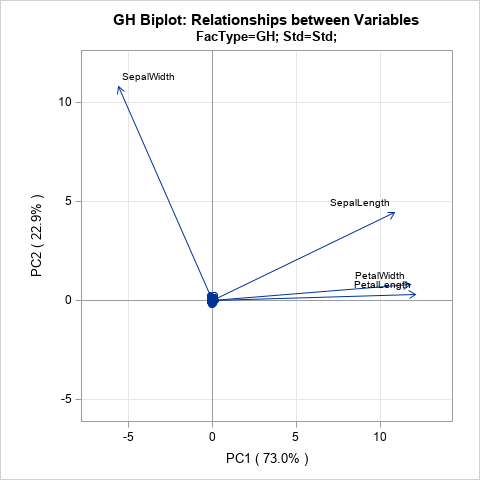If you choose c = 0, then A = U and B = L V`. The literature calls this biplot the GH biplot. I call it the "variable preserving" biplot because it provides the most faithful two-dimensional representation of the relationship between vectors. In particular:

• The length of each vector (a row of B) is proportional to the variance of the corresponding variable.
• The Euclidean distance between the i_th and j_th rows of A is proportional to the Mahalanobis distance between the i_th and j_th observations in the data.

In preserving the lengths of the vectors, this biplot distorts the Euclidean distance between points. However, the distortion is not arbitrary: it represents the Mahalanobis distance between points.

The GH biplot is shown to the right, but it is not very useful for these data. In choosing to preserve the variable relationships, the observations are projected onto a tiny region near the origin. The next section discusses an alternative scaling that is more useful for the iris data.

### The COV biplot

If you choose c = 0 and β = sqrt(N-1), then A = sqrt(N-1) U and B = L V` / sqrt(N-1). The literature calls this biplot the COV biplot. This biplot is shown at the top of this article. It has two useful properties:

• The length of each vector is equal to the variance of the corresponding variable.
• The Euclidean distance between the i_th and j_th rows of A is equal to the Mahalanobis distance between the i_th and j_th observations in the data.

In my opinion, the COV biplot is usually superior to the GH biplot.

### The JK biplot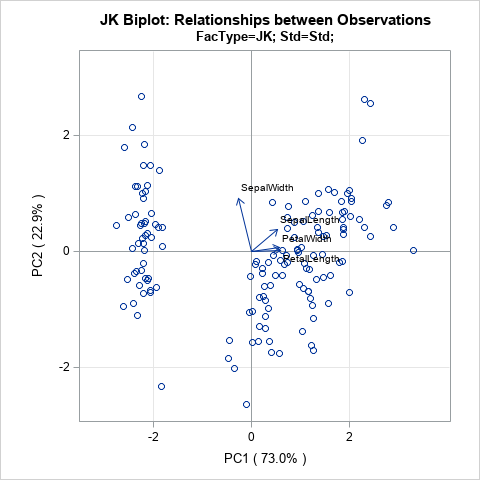If you choose c = 1, you get the JK biplot, which preserves the Euclidean distance between observations. Specifically, the Euclidean distance between the i_th and j_th rows of A is equal to the Euclidean distance between the i_th and j_th observations in the data.

In faithfully representing the observations, the angles between vectors are distorted by the scaling.

### The SYM biplot

If you choose c = 1/2, you get the SYM biplot (also called the SQ biplot), which attempts to treat observations and variables in a symmetric manner. Although neither the observations nor the vectors are faithfully represented, often neither representation is very distorted. Consequently, some people prefer the SYM biplot as a compromise between the COV and JK biplots. The SYM biplot is shown in the next section.

### How to interpret a biplot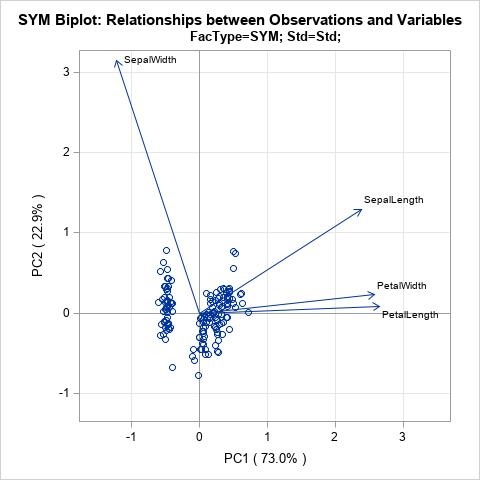As discussed in the SAS/IML Studio User's Guide, you can interpret a biplot in the following ways:

• The cosine of the angle between a vector and an axis indicates the importance of the contribution of the corresponding variable to the principal component.
• The cosine of the angle between pairs of vectors indicates correlation between the corresponding variables. Highly correlated variables point in similar directions; uncorrelated variables are nearly perpendicular to each other.
• Points that are close to each other in the biplot represent observations with similar values.
• You can approximate the relative coordinates of an observation by projecting the point onto the variable vectors within the biplot. However, you cannot use these biplots to estimate the exact coordinates because the vectors have been centered and scaled. You could extend the vectors to become lines and add tick marks, but that becomes messy if you have more than a few variables.

If you want to faithfully interpret the angles between vectors, you should equate the horizontal and vertical axes of the biplot, as I have done with the plots on this page.

If you apply these facts to the standardized iris data, you can make the following interpretations:

• The PetalLength and PetalWidth variables are the most important contributors to the first PC. The SepalWidth variable is the most important contributor to the second PC.
• The PetalLength and PetalWidth variables are highly correlated. The SepalWidth variable is almost uncorrelated with the other variables.
• Although I have suppressed labels on the points, you could label the points by an ID variable or by the observation number and use the relative locations to determine which flowers had measurements that were most similar to each other.

### Summary

This article presents an overview of biplots. A biplot is an overlay of a score plot and a loadings plot, which are two common plots in a principal component analysis. These two plots are on different scales, but you can rescale the two plots and overlay them on a single plot. Depending upon the choice of scaling, the biplot can provide faithful information about the relationship between variables (lengths and angles) or between observations (distances). It can also provide approximates relationships between variables and observations.

In a subsequent post, I will show how to use SAS to create the biplots in this article.

The post What are biplots? appeared first on The DO Loop.In response to a recent article about how to compute the cosine similarity of observations, a reader asked whether it is practical (or even possible) to perform these types of computations on data sets that have many thousands of observations. The problem is that the cosine similarity matrix is an N x N matrix, where N is the sample size. Thus, the matrix can be very big even for moderately sized data.

The same problem exists for all distance- and similarity-based measurements. Because the Euclidean distance is familiar to most readers, I will use distance to illustrate the techniques in this article. To be specific, suppose you have N=50,000 observations. The full distance matrix contains 2.5 billion distances between pairs of observations. However, in many applications, you are not interested in all the distances. Rather, you are interested in extreme distances, such as finding observations that are very close to each other or are very far from each other. For definiteness, suppose you want to find observations that are less than a certain distance from each other. That distance (called the cutoff distance) serves as a filter. Instead of storing N2 observations, you only need to store the observations that satisfy the cutoff criterion.

### Compute by using block-matrix computations

A way to perform a distance computation is to divide the data into blocks and perform block-matrix computations. If X is the data matrix, then the pairwise distance matrix of the N rows is an N x N matrix. But you can subdivide X into blocks where each block has approximately M rows, where M is a smaller number such as M=1,000. Write X = [A1, A2, ..., Ak], where the block matrices are stacked vertically. Then the full distance matrix D = dist(X, X) is equal to stacking the distance matrices for each of the block matrices: D = [dist(A1,X), dist(A2,X), ..., dist(Ak,X)]. Each of the block matrices is an M x N matrix. The following diagram shows the block-matrices. The main idea is to compute each block matrix (which fits into memory), then identify and extract the values that satisfy the cutoff distance. With this technique, you never hold the full N x N distance matrix in memory.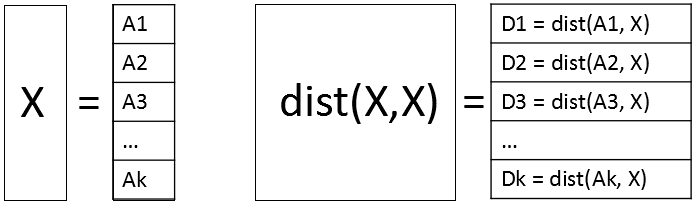### A small example of block computations

A programming principle that I strongly endorse is "start small." Even if your goal is to work with large data, develop and debug the program for small data. In this section, the data contains only 13 observations and 10 variables. The goal is to use block-matrix computations (with a block size of M=3) to compute the 132 = 169 pairwise distances between the observations. As each block of distances is computed, those distances that are less than 2 (the cutoff distance in this example) are identified and stored. The data are simulated by using the following DATA step:

```/* start with a smaller example: 13 observations and 10 vars */ %let N = 13; data Have; call streaminit(12345); array x; do i = 1 to &N; do j = 1 to dim(x); x[j] = rand("Uniform", -1, 1); end; output; end; keep x:; run;```

It makes sense to report the results by giving the pair of observations numbers and the distance for those pairs that satisfy the criterion. For these simulated data, there are 10 pairs of observations that are closer than 2 units apart, as we shall see shortly.

The following SAS/IML program shows how to use block computation to compute the pairs of observations that are close to each other. For illustration, set the block size to M=3. Suppose you are computing the second block, which contains the observations 4, 5, and 6. The following program computes the distance matrix from those observations to every other observation:

```proc iml; use Have; read all var _NUM_ into X; close; m = 3; /* block size */ n = nrow(X); /* number of observations */ cutoff = 2; /* report pairs where distance < cutoff */   /* Block matrix X = [A1, A2, A3, ..., Ak] */ startrow=4; endrow=6; /* Example: Look at 2nd block with obs 4,5,6 */ rows = startRow:endRow;   /* Compute D_i = distance(Ai, X) */ A = X[rows,]; /* extract block */ D = distance(A, X); /* compute all distances for obs in block */ print D[r=rows c=(1:n) F=5.2];```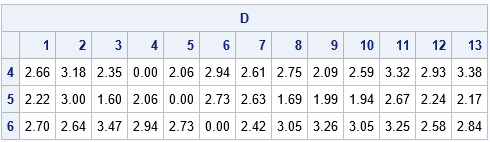The output shows the distances between the 4th, 5th, and 6th observations and all other observation. From the output, you can see that

• The 4th observation is not less than 2 units from any observation (except itself).
• The 5th observation is within 2 units of observations 3, 8, 9, and 10.
• The 6th observation is not less than 2 units from any observation.

The following statements use the LOC function to locate the elements of D that satisfy the cutoff criterion. (You should always check that the vector returned by the LOC function is nonempty before you use it.) The NDX2SUBS function converts indices into (row, col) subscripts. The subscripts are for the smaller block matrix, so you have to map them to the row numbers in the full distance matrix:

```/* Extract elements that satisfy the criterion */ idx = loc( D < cutoff ); /* find elements that satisfy cutoff criterion */ *if ncol(idx) > 0 then do; /* should check that idx is not empty */ Distance = D[idx]; /* extract close distances */ s = ndx2sub(dimension(D), idx); /* s contains matrix subscripts */ Row = rows[s[,1]]; /* convert rows to row numbers for full matrix */ Col = s[,2]; print Row Col Distance[F=5.2];```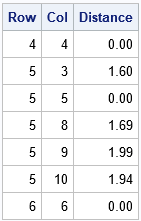Because the distance matrix is symmetric (and we can exclude the diagonal elements, which are 0), let's agree to report only the upper-triangular portion of the (full) distance matrix. Again, you can use the LOC function to find the (Row, Col) pairs for which Row is strictly less than Col. Those are the pairs of observations that we want to store:

``` /* filter: output only upper triangular when full matrix is symmetric */ k = loc( Row < Col ); /* store only upper triangular in full */ *if ncol(k)>0 then do; /* should check that k is not empty */ Row = Row[k]; Col = Col[k]; Distance = Distance[k]; print Row Col Distance[F=5.2];```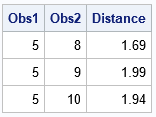Success! The printed observations are the elements of the upper-triangular distance matrix that satisfy the cutoff criterion. If you repeat these computations for other blocks (observations 1-3, 4-6, 7-9, 10-12, and 13) then you can compute all pairs of observations that are less than 2 units from each other. For the record, here are the 10 pairs of observations that are within 0.5 units of each other. Although the point of this article is to avoid computing the full 13 x 13 distance matrix, for this small example you can check the following table by visual inspection of the full distance matrix, F = distance(X, X).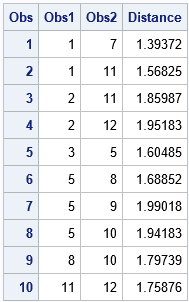### Block computations to compute distances in large data

The previous section describes the main ideas about using block matrix computations to perform distance computations for large data when the whole N x N distance matrix will not fit into memory. The same ideas apply to cosine similarity and or other pairwise computations. For various reasons, I recommend that the size of the block matrices be less than 2 GB.

The following DATA step generates 50,000 observations, which means there are 2.5 billion pairs of observations. The program computes all pairwise distances and finds all pairs that are within 0.5 units. The full distance matrix is about 18.6 GB, but the program never computes the full matrix. Instead, the program uses a block size of 5,000 rows and computes a total of 10 distance matrices, each of which requires 1.86 GB of RAM. (See "How much RAM do I need to store that matrix?) For each of these block matrices, the program finds and writes the pairs of observations that are closer than 0.5 units. The program requires about a minute to run on my desktop PC. If you have a slow PC, you might want to use N=20000 to run the example.

```/* Create 10 variables and a large number (N) of obs. The complete distance matrix requires N^2 elements. */ %let N = 50000; data Have; call streaminit(12345); array x; do i = 1 to &N; do j = 1 to dim(x); x[j] = rand("Uniform", -1, 1); end; output; end; keep x:; run;   /* Find pairs of observations that within 'cutoff' of each other. Write these to a data set. */ proc iml; use Have; read all var _NUM_ into X; close; n = nrow(X); /* number of observations */ m = 5000; /* block size: best if n*m elements fit into 2 GB RAM */ cutoff = 0.5; /* report pairs where distance < cutoff */   startRow = 1; /* set up output data set to store results */ create Results var {'Obs1' 'Obs2' 'Distance'}; do while (startRow <= n); /* Block matrix X = [A1, A2, A3, ..., Ak] */ endRow = min(startRow + m - 1, n); /* don't exceed size of matrix */ rows = startRow:endRow; /* Compute D_i = distance(Ai, X) */ A = X[rows,]; /* extract block */ D = distance(A, X); /* compute all distances for obs in block */   /* Extract elements that satisfy the criterion */ idx = loc( D < cutoff ); /* find elements that satisfy cutoff criterion */ if ncol(idx) > 0 then do; Distance = D[idx]; /* extract close distances */ s = ndx2sub(dimension(D), idx); /* s contains matrix subscripts */ Row = rows[s[,1]]; /* convert rows to row numbers for full matrix */ Col = s[,2]; /* filter: output only upper triangular when full matrix is symmetric */ k = loc( Row < Col ); /* store only upper triangular in full */ if ncol(k)>0 then do; Obs1 = Row[k]; Obs2 = Col[k]; Distance = Distance[k]; append; /* Write results to data set */ end; end; startRow = startRow + m; end; close; QUIT;   title "Distances Less than 0.5 for Pairs of Observations"; title2 "N = 50,000"; proc sgplot data=Results; histogram Distance; run;```

From the 2.5 billion distances between pairs of observations, the program found 1620 pairs that are closer than 0.5 units in a 10-dimensional Euclidean space. The distribution of those distances is shown in the following histogram.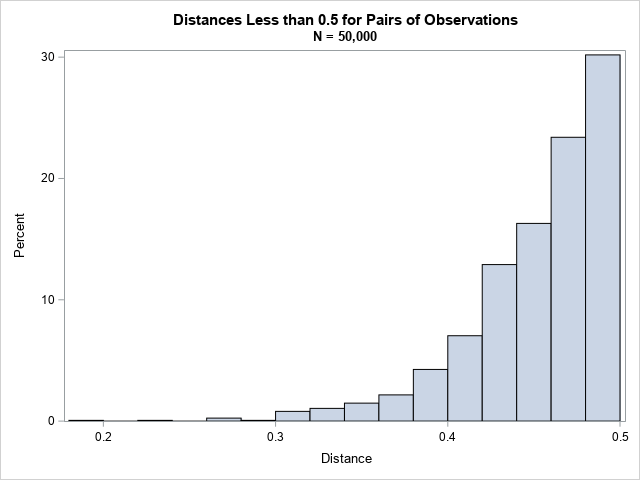### Further improvements

When the full matrix is symmetric, as in this example, you can improve the algorithm. Because only the upper triangular elements are relevant, you can improve the efficiency of the algorithm by skipping computations that are in the lower triangular portion of the distance matrix.

The k_th block matrix, A, represents consecutive rows in the matrix X. Let L be the row number of the first row of X that is contained in A. You do not need to compute the distance between rows of A and all rows of X. Instead, set Y = X[L:N, ] and compute distance(A, Y). With this modification, you need additional bookkeeping to map the column numbers in the block computation to the column numbers in the full matrix. However, for large data sets, you decrease the computational time by almost half. I leave this improvement as an exercise for the motivated reader.

### Conclusions

In summary, you can use a matrix langue like SAS/IML to find pairs of observations that are close to (or far from) each other. By using block-matrix computations, you never have to compute the full N x N distance matrix. Instead, you can compute smaller pieces of the matrix and find the pairs of observations that satisfy your search criterion.

Because this problem is quadratic in the number of observations, you can expect the program to take four times as long if you double the size of the input data. So the program in this article will take about four minutes for 100,000 observations and 16 minutes for 200,000 computations. If that seems long, remember that with 200,000 observations, the program must compute 40 billion pairwise distances.

The post Perform matrix computations when the matrices don't fit in memory appeared first on The DO Loop.For linear regression models, there is a class of statistics that I call deletion diagnostics or leave-one-out statistics. These observation-wise statistics address the question, "If I delete the i_th observation and refit the model, what happens to the statistics for the model?" For example:

• The PRESS statistic is similar to the residual sum of squares statistic but is based on fitting n different models, where n is the sample size and the i_th model excludes the i_th observation.
• Cook's D statistic measures the influence of the i_th observation on the fit.
• The DFBETAS statistics measure how the regression estimates change if you delete the i_th observation.

Although most references define these statistics in terms of deleting an observation and refitting the model, you can use a mathematical trick to compute the statistics without ever refitting the model! For example, the Wikipedia page on the PRESS statistic states, "each observation in turn is removed and the model is refitted using the remaining observations. The out-of-sample predicted value is calculated for the omitted observation in each case, and the PRESS statistic is calculated as the sum of the squares of all the resulting prediction errors." Although this paragraph is conceptually correct, theSAS/STAT documentation for PROC GLMSELECT states that the PRESS statistic "can be efficiently obtained without refitting the model n times."

### A rank-1 update to the inverse of a matrix

Recall that you can use the "normal equations" to obtain the least squares estimate for the regression problem with design matrix X and observed responses Y. The normal equations are b = (X`X)-1(X`Y), where X`X is known as the sum of squares and crossproducts (SSCP) matrix and b is the least squares estimate of the regression coefficients. For data sets with many observations (very large n), the process of reading the data and forming the SSCP is a relatively expensive part of fitting a regression model. Therefore, if you want the PRESS statistic, it is better to avoid rebuilding the SSCP matrix and computing its inverse n times. Fortunately, there is a beautiful result in linear algebra that relates the inverse of the full SSCP matrix to the inverse when a row of X is deleted. The result is known as the Sherman-Morrison formula for rank-1 updates.

The key insight is that one way to compute the SSCP matrix is as a sum of outer products of the rows of X. Therefore if xi is the i_th row of X, the SCCP matrix for data where xi is excluded is equal to X`X - xi`xi. You have to invert this matrix to find the least squares estimates after excluding xi.

The Sherman-Morrison formula enables you to compute the inverse of X`X - xi`xi when you already know the inverse of X`X. For brevity, set A = X`X. The Sherman-Morrison formula for deleting a row vector xi` is
(A – xi`xi)-1 = A-1 + A-1 xi`xi A-1 / (1 – xiA-1xi`)

### Implement the Sherman-Morrison formula in SAS

The formula shows how to compute the inverse of the updated SSCP by using a matrix-vector multiplication and an outer product. Let's use a matrix language to demonstrate the update method. The following SAS/IML program reads in a small data set, forms the SSCP matrix (X`X), and computes its inverse:

```proc iml; use Sashelp.Class; /* read data into design matrix X */ read all var _NUM_ into X[c=varNames]; close; XpX = X`*X; /* form SSCP */ XpXinv = inv(XpX); /* compute the inverse */```

Suppose you want to compute a leave-one-out statistic such as PRESS. For each observation, you need to estimate the parameters that result if you delete that observation. For simplicity, let's just look at deleting the first row of the X matrix. The following program creates a new design matrix (Z) that excludes the row, forms the new SSCP matrix, and finds its inverse:

```/* Inefficient: Manually delete the row from the X matrix and recompute the inverse */ n = nrow(X); Z = X[2:n, ]; /* delete first row */ ZpZ = Z`*Z; /* reform the SSCP matrix */ ZpZinv = inv(ZpZ); /* recompute the inverse */ print ZpZinv[c=varNames r=varNames L="Inverse of SSCP After Deleting First Row"];```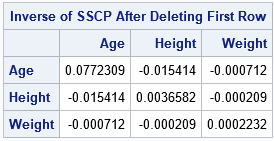The previous statements essentially repeat the entire least squares computation. To compute a leave-one-out statistic, you would perform a total of n similar computations.

In contrast, it is much cheaper to apply the Sherman-Morrison formula to update the inverse of the original SSCP. The following statements apply the Sherman-Morrison formula as it is written:

```/* Alternative: Do not change X or recompute the inverse. Use the Sherman-Morrison rank-1 update formula. https://en.wikipedia.org/wiki/Sherman–Morrison_formula */ r = X[1, ]; /* first row */ rpr = r`*r; /* outer product */ /* apply Sherman-Morrison formula */ NewInv = XpXinv + XPXinv*rpr*XPXinv / (1 - r*XpXinv*r`); print NewInv[c=varNames r=varNames L="Inverse from Sherman-Morrison Formula"];```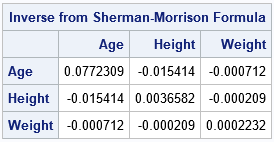These statements compute the new inverse by using the old inverse, an outer product, and a few matrix multiplications. Notice that the denominator of the Sherman-Morrison formula includes the expression r*(X`X)-1*r`, which is the leverage statistic for the i_th row.

### The INVUPDT function in SAS/IML

Because it is important to be able to update an inverse matrix quickly when an observation is deleted (or added!), the SAS/IML language supports the IMVUPDT function, which implements the Sherman-Morrison formula. You merely specify the inverse matrix to update, the vector (as a column vector) to use for the rank-one update, and an optional scalar value, which is usually +1 if you are adding a new observation and -1 if you are deleting an observation. For example, the following statements are the easiest way to implement the Sherman-Morrison formula in SAS for a leave-one-out statistic:

```NewInv2 = invupdt(XpXinv, r`, -1); print NewInv2[c=varNames r=varNames L="Inverse from INVUPDT Function"];```

The output is not displayed because the matrix NewInv2 is the same as the matrix NewInv in the previous section. The documentation includes additional examples.

### The general Sherman-Morrison-Woodbury formula

The Sherman-Morrison formula shows how to perform a rank-1 update of an inverse matrix. There is a more general formula, called the Sherman-Morrison-Woodbury formula, which enables you to update an inverse for any rank-k modification of the original matrix. The general formula (Golub and van Loan, p. 51 of 2nd ed. or p. 65 of 4th ed.) shows how to find the matrix of a rank-k modification to a nonsingular matrix, A, in terms of the inverse of A. The general formula is
(A + U VT)-1 = A-1 – A-1 U (I + VT A-1 U) VT A-1
where U and V are p x k and all inverses are assumed to exist. When k = 1, the matrices U and V become vectors and the k x k identify matrix becomes the scalar value 1. In the previous section, U equals -xiT and V equals xiT.

The Sherman-Morrison-Woodbury formula is one of my favorite results in linear algebra. It shows that a rank-k modification of a matrix results in a rank-k modification of its inverse. It is not only a beautiful theoretical result, but it has practical applications to leave-one-out statistics because you can use the formula to quickly compute the linear regression model that results by dropping an observation from the data. In this way, you can study the influence of each observation on the model fit (Cook's D, DFBETAS,...) and perform leave-one-out cross-validation techniques, such as the PRESS statistic.

The post Leave-one-out statistics and a formula to update a matrix inverse appeared first on The DO Loop.